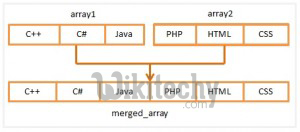# php tutorial - How to Merge Arrays in PHP - php programming - learn php - php code - php script

• PHP provides some built-in array functions which help to do array operation so easily. The array_merge() function is one of them.Learn PHP Tutorial - PHP Tutorial tutorial - merge array in php - PHP Tutorial examples - PHP Tutorial programs

• PHP array_merge() function creates a new array merging two or more PHP arrays but the original arrays remain unchanged.
• The structure of a array_merge() function is given below…
• The structure shows that the array_merge() function accepts two or more arrays as arguments and returns another array as a return value but does not change the original arrays.

## Output

• The example shows that two arrays are merging with the array_merge() function but the original arrays are remaining unchanged.
• So, multiple PHP arrays can easily be merged using the array_merge() function.

## PHP array_combine() function

• The array_combine() function helps to create a new array combining two arrays where one array elements will be indexes and another array elements will be elements of the new array.
• But the both arrays must have equal elements.

## Output

• The example shows that the array_combine() function returns an array which indexes are the first array elements and the values are the second array elements.
• So, two PHP arrays can be combined using the array_combine() function so easily.